# RD Sharma Solutions Class 12 The Plane Exercise 29.6

RD Sharma Solutions for Class 12 Maths Chapter 29 The Plane Exercise 29.6, is given here. Experts have designed RD Sharma Solutions in a very lucid manner that helps students solve problems in the most efficient possible ways. Students are advised to practice the RD Sharma Class 12 Solutions regularly to achieve their desired scores. Students can easily download the pdf consisting of this chapter solutions, which are available in the links provided below and can use it for future reference as well.

## Download PDF of RD Sharma Solutions For Class 12 Maths Chapter 29 Exercise 6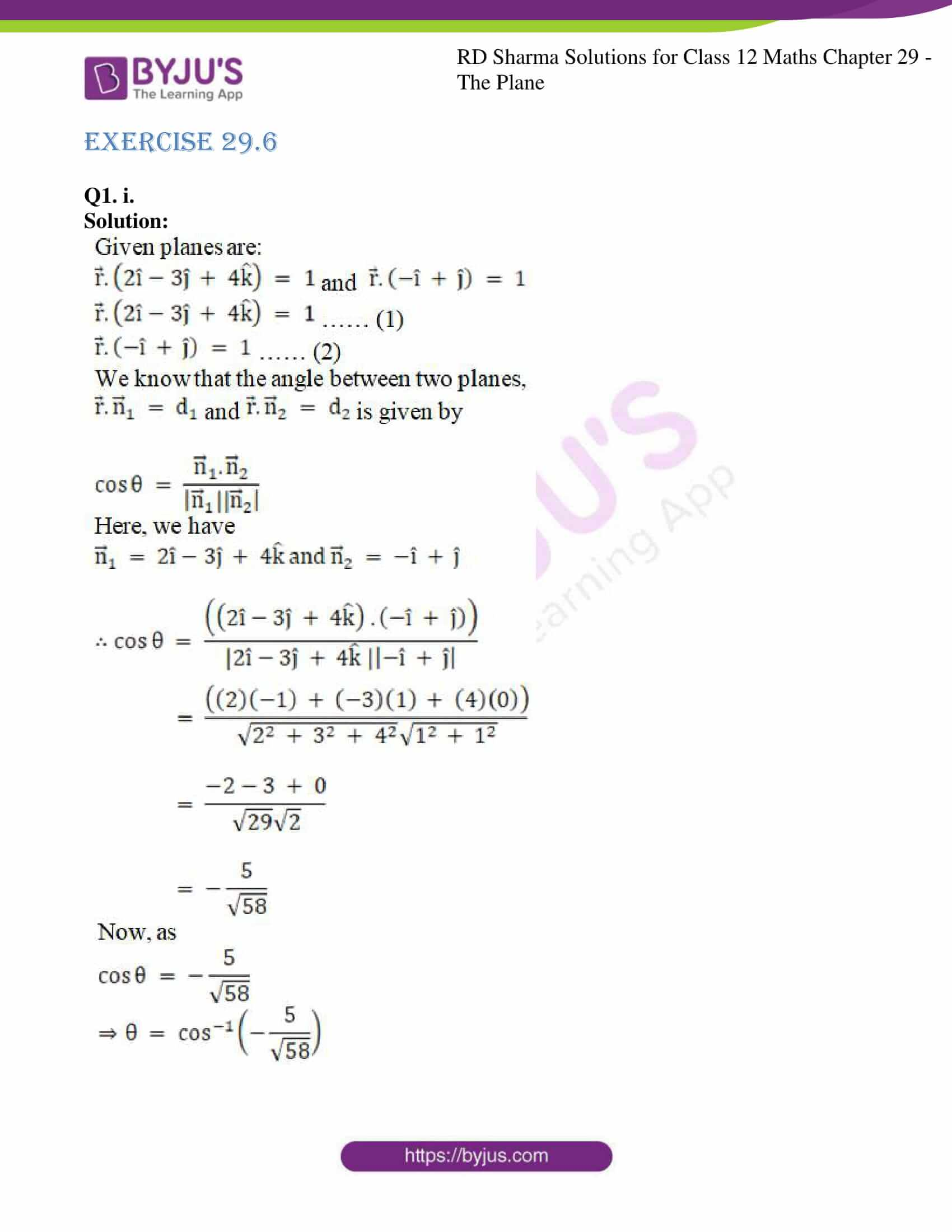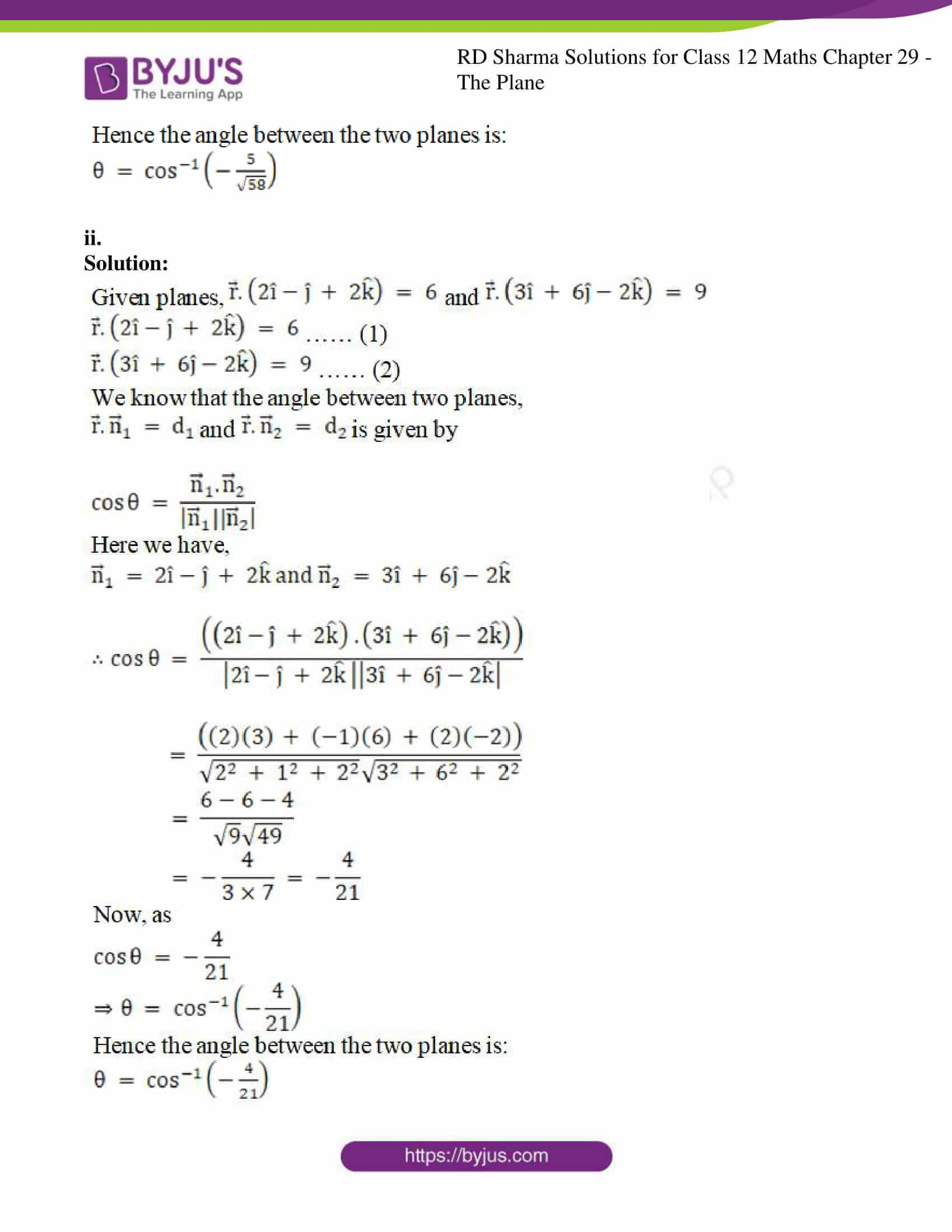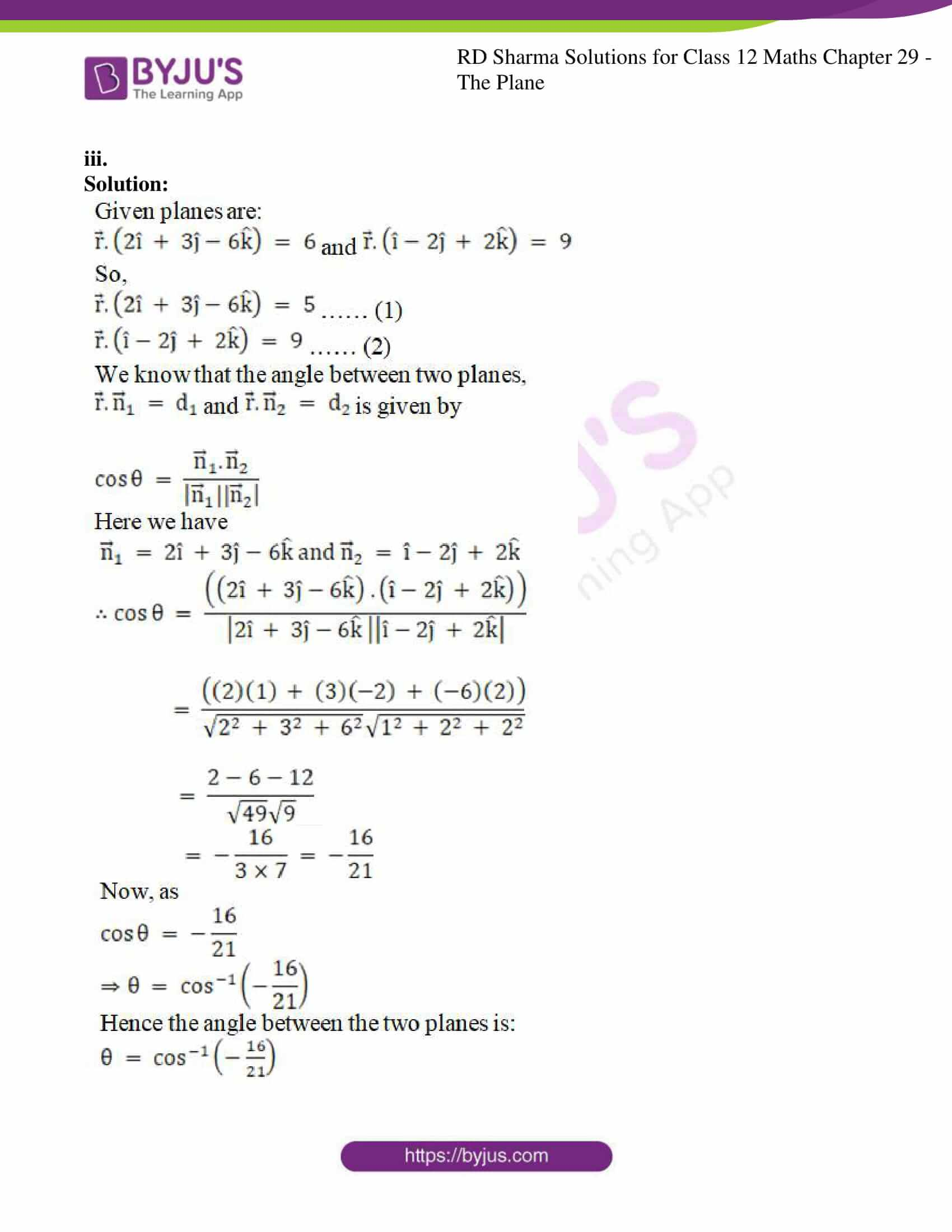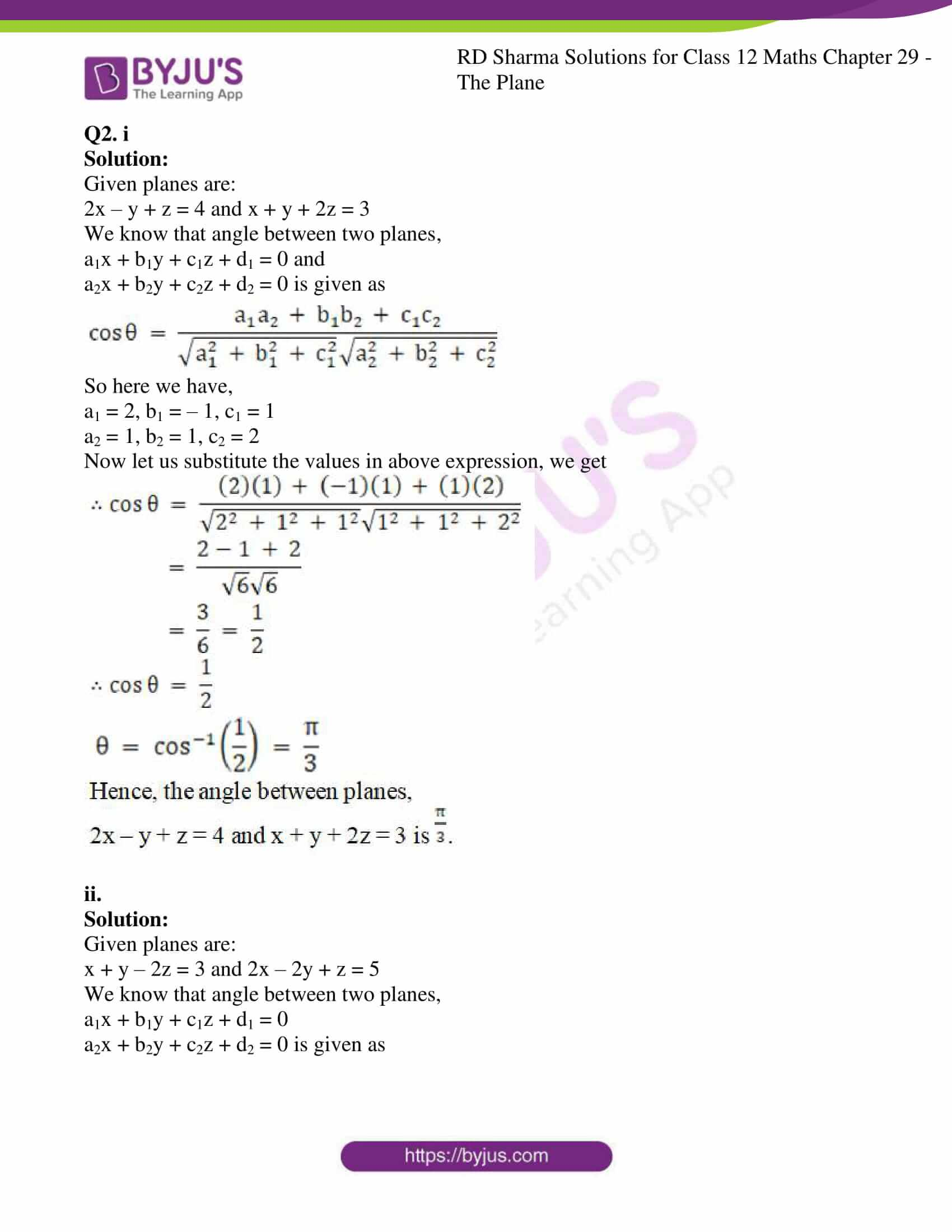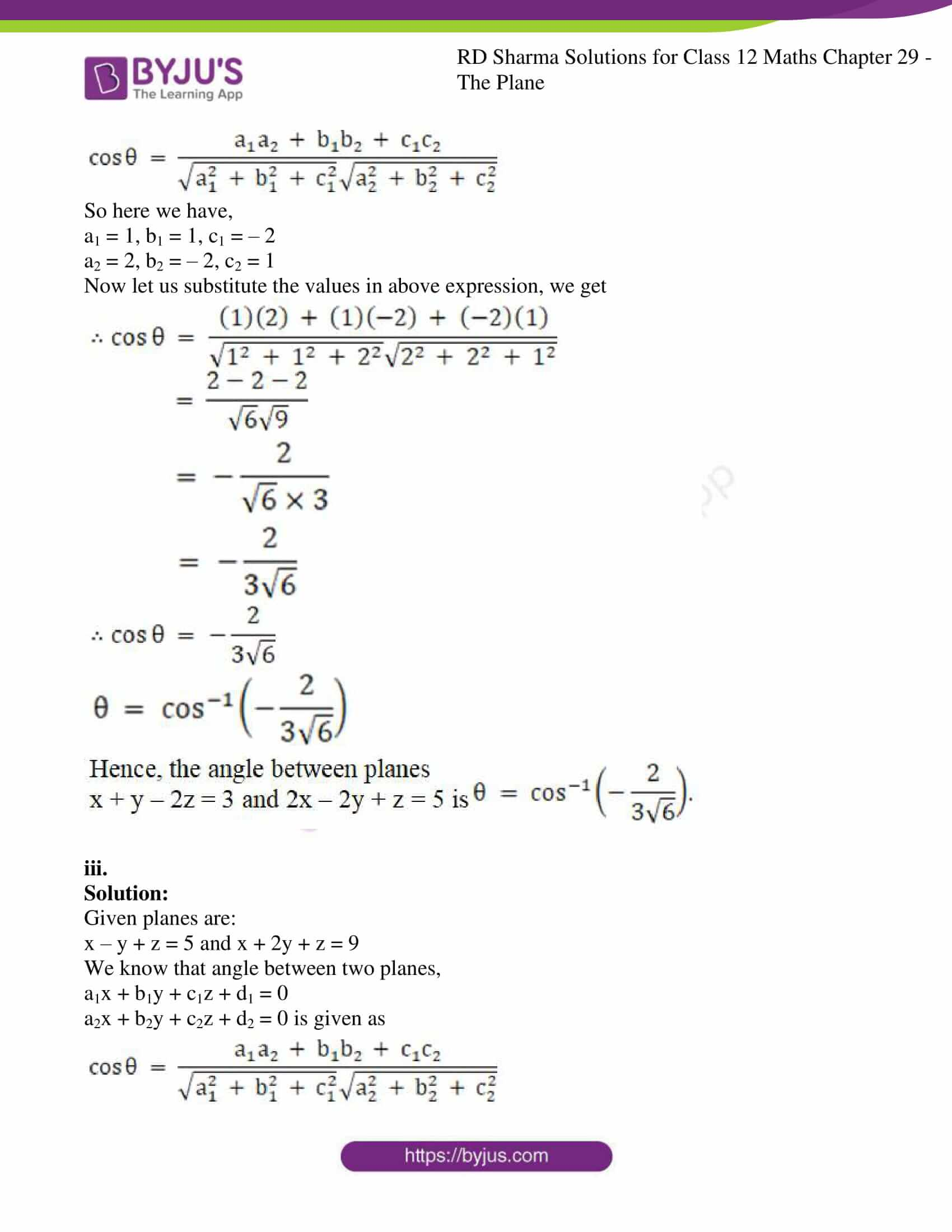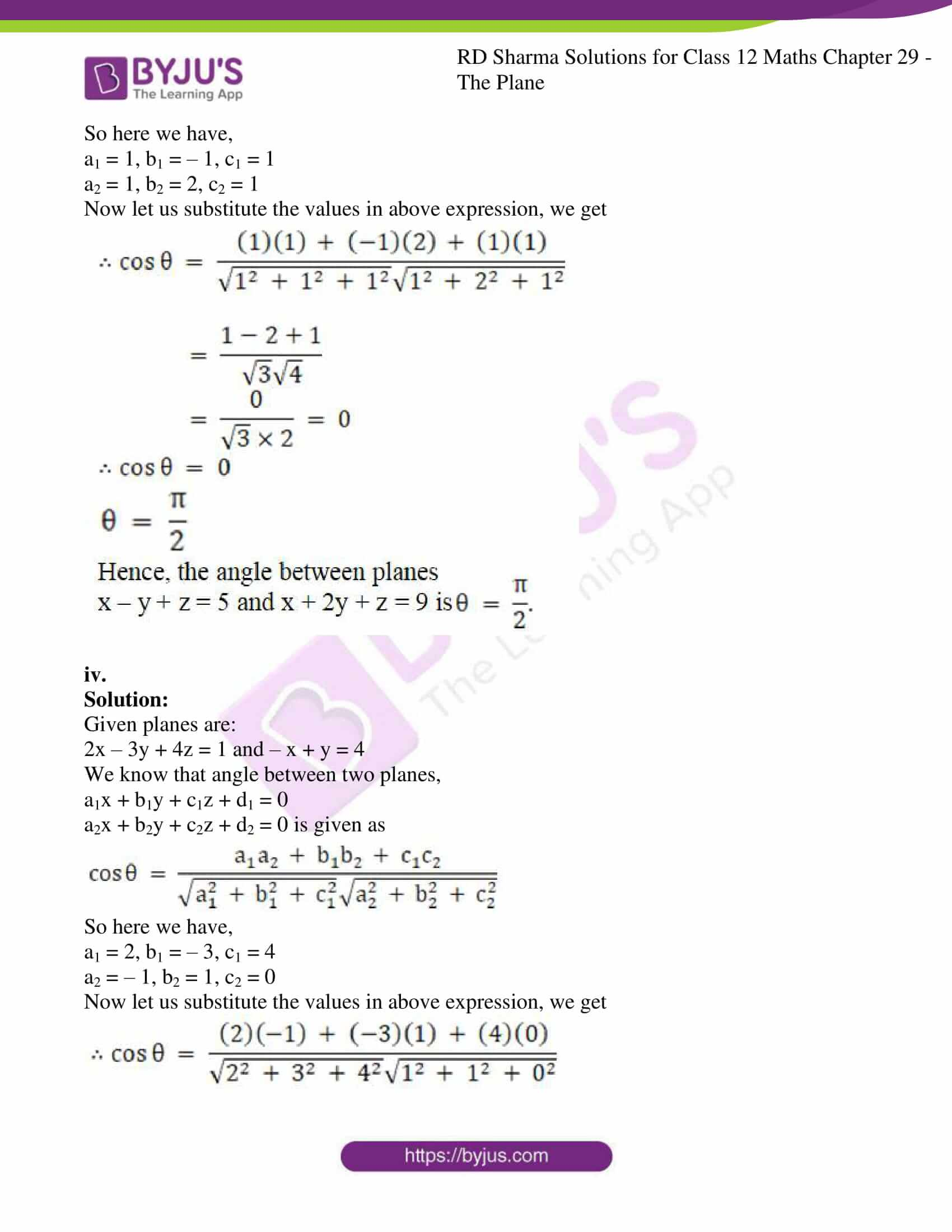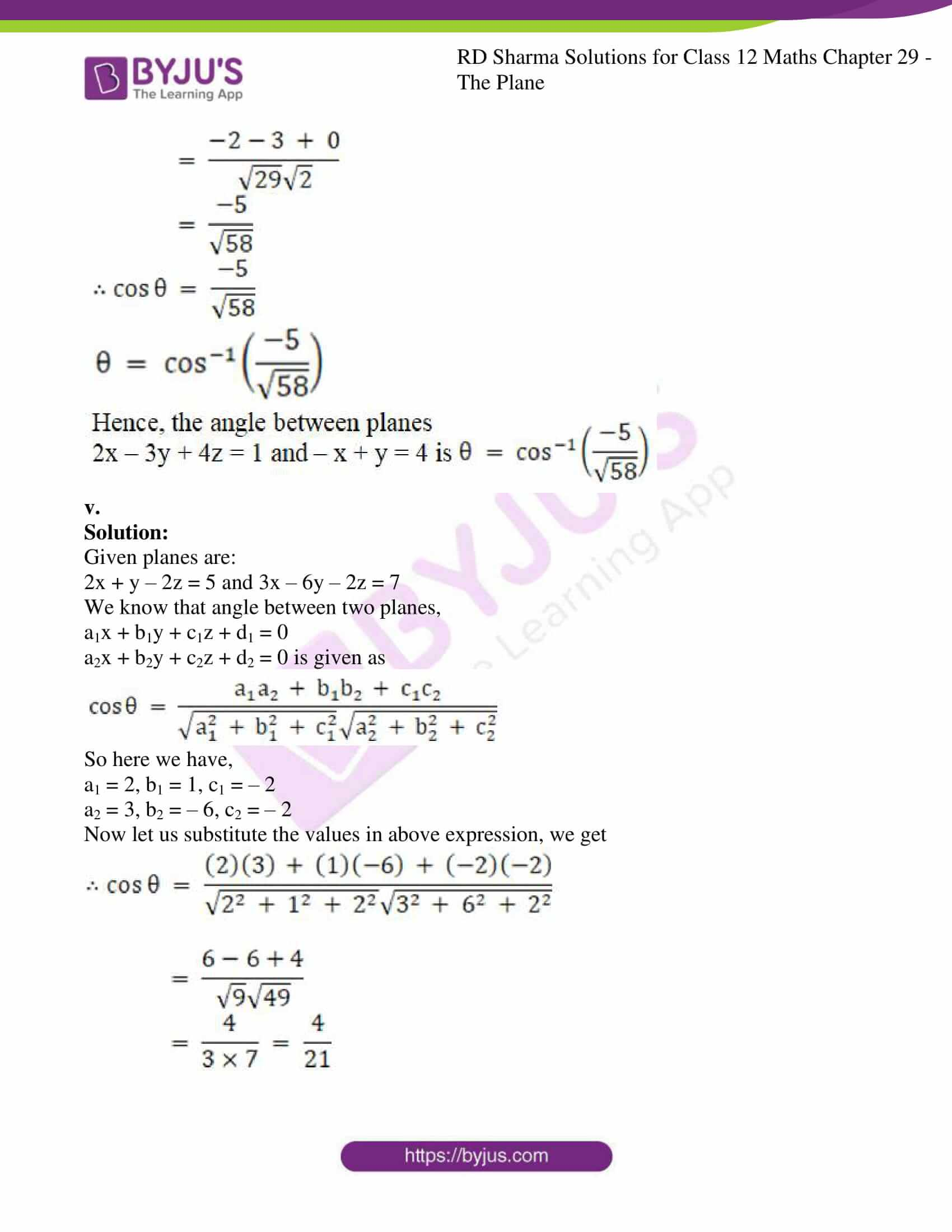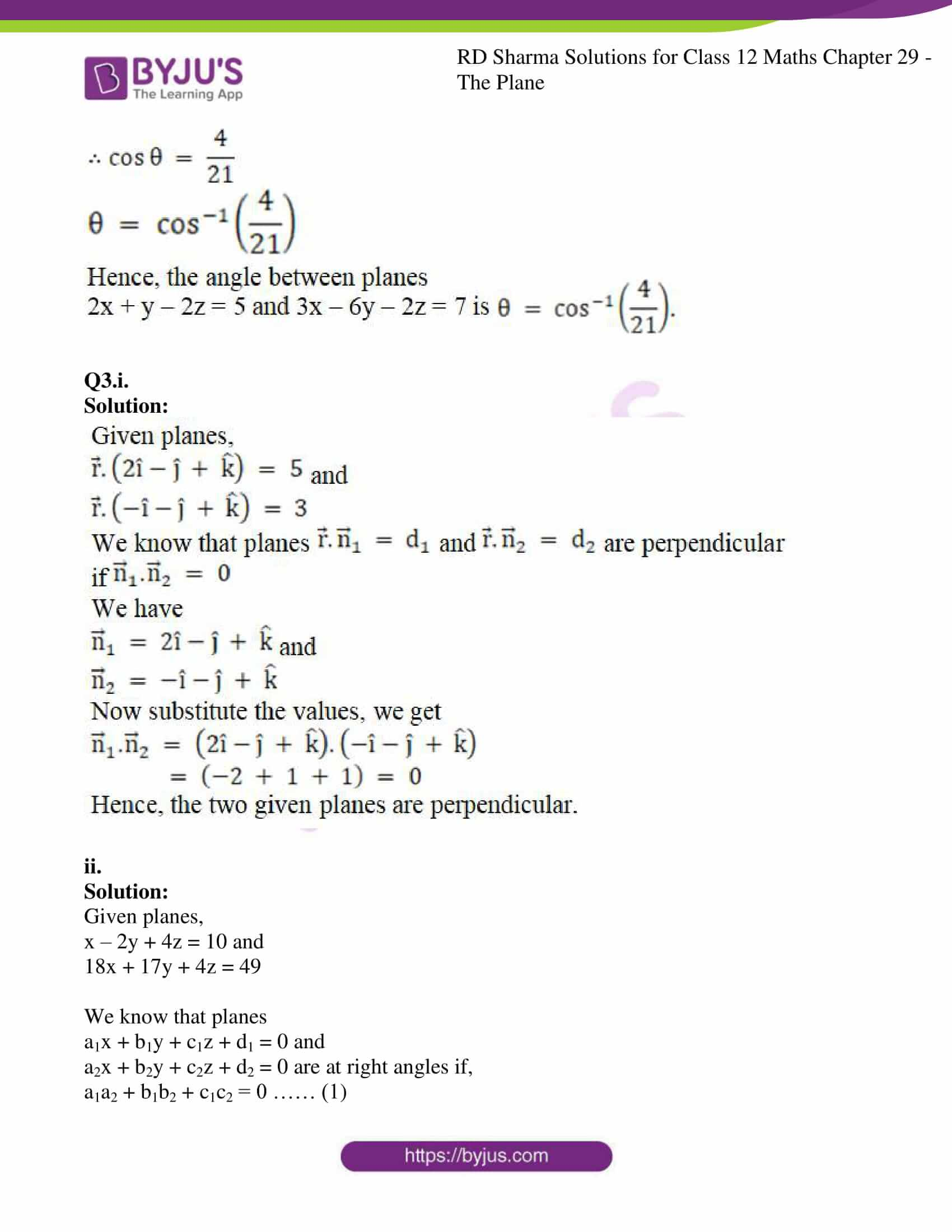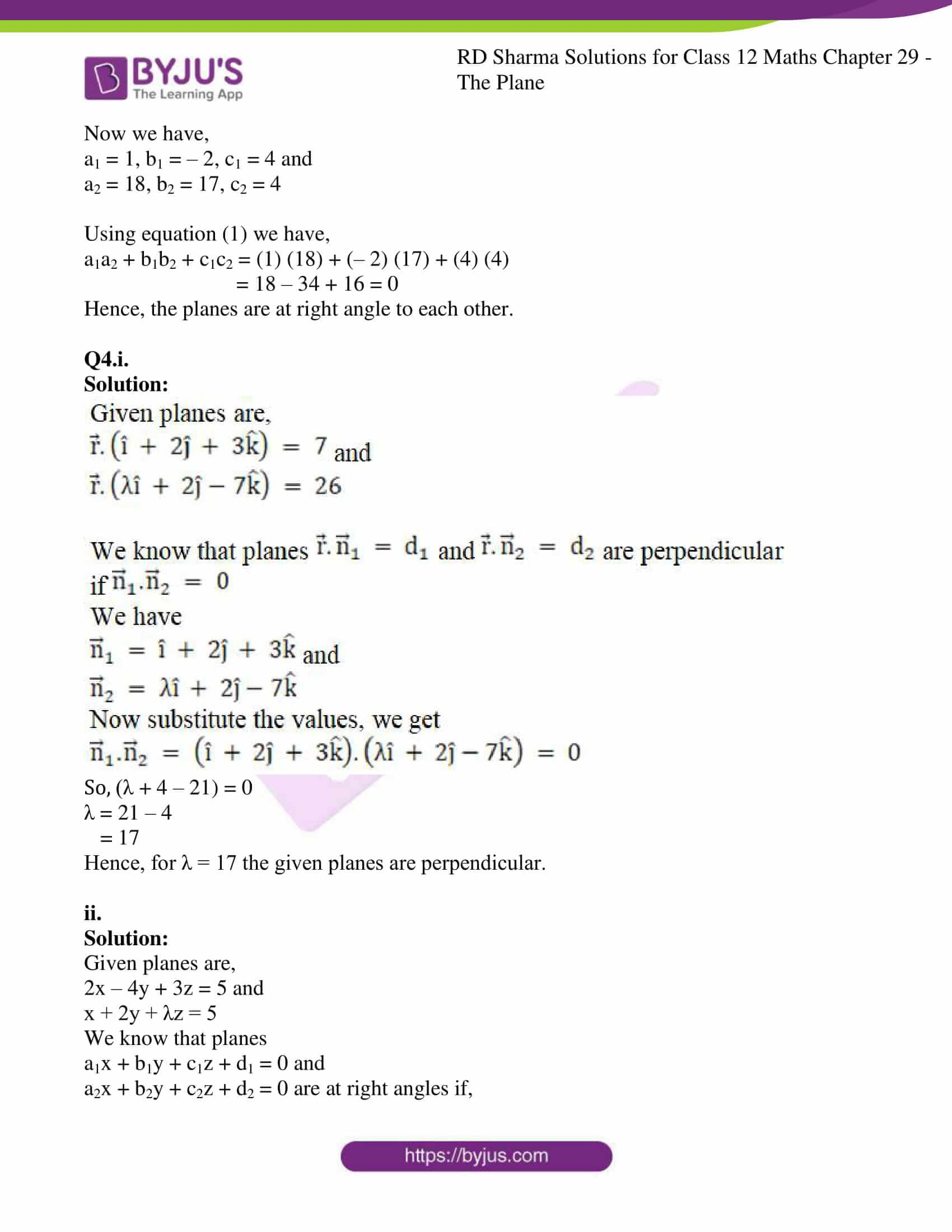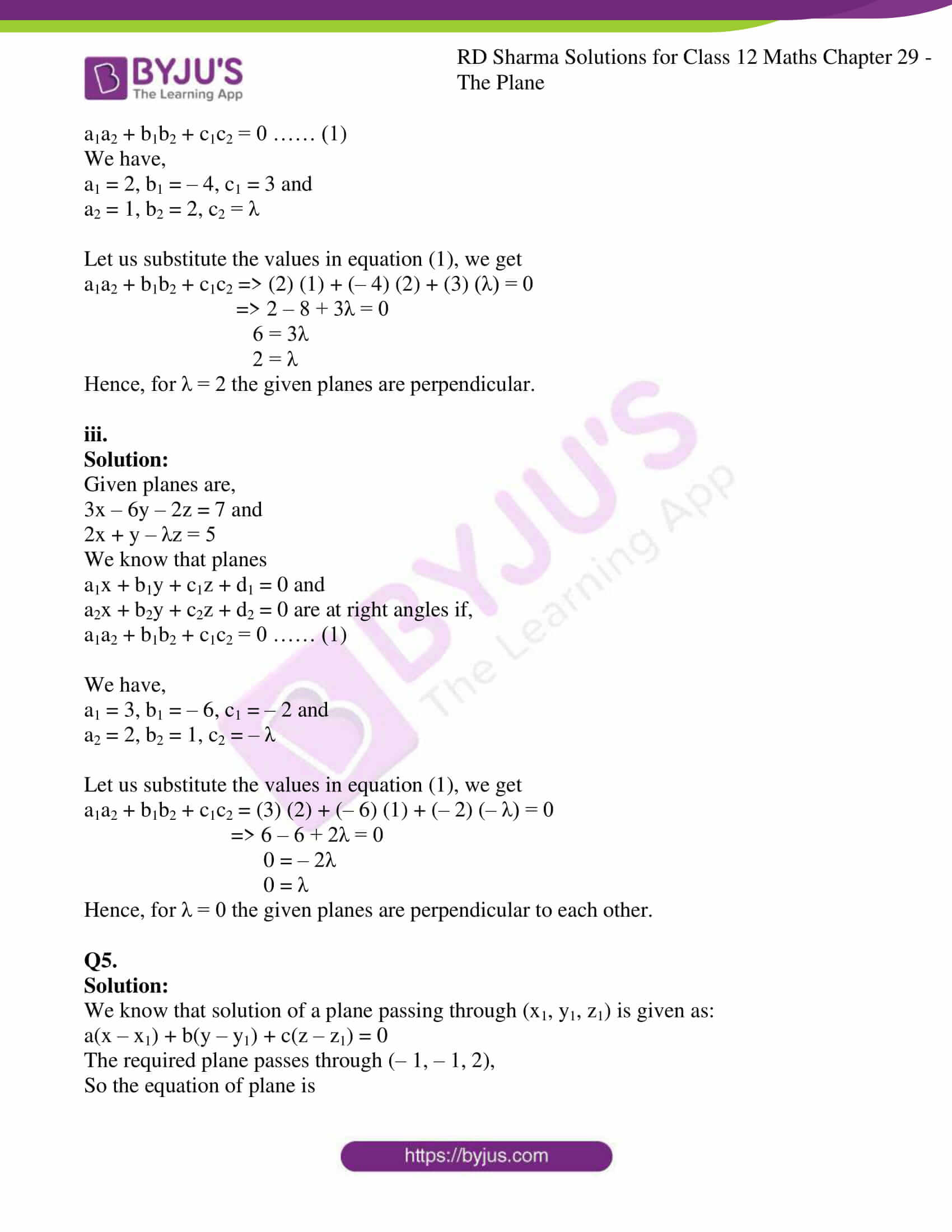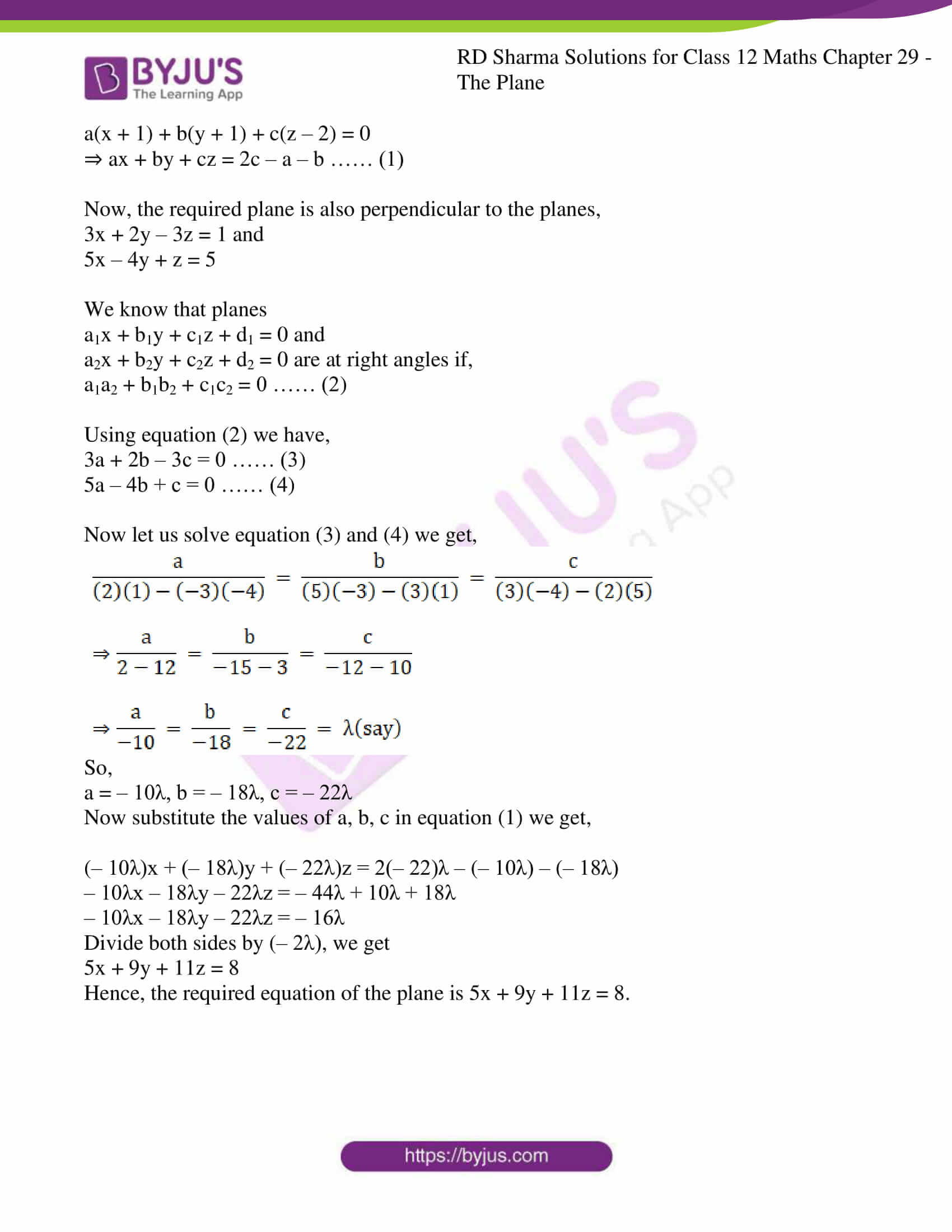### Access RD Sharma Solutions For Class 12 Maths Chapter 29 Exercise 6

EXERCISE 29.6

Q1. i.

Solution: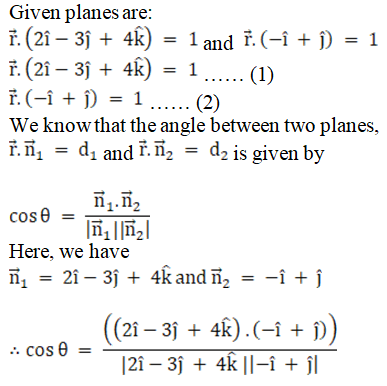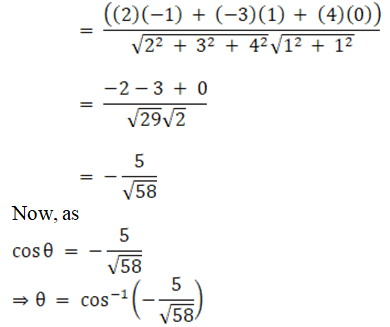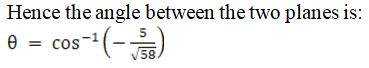ii.

Solution: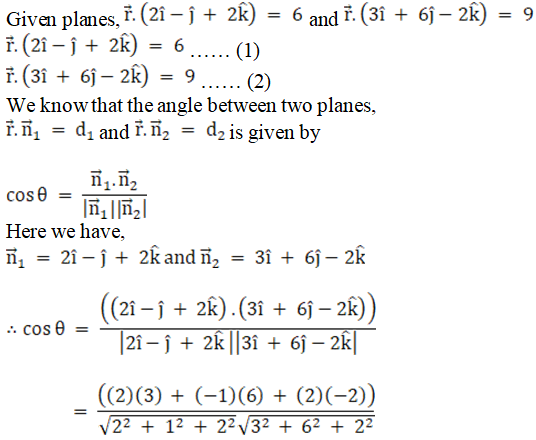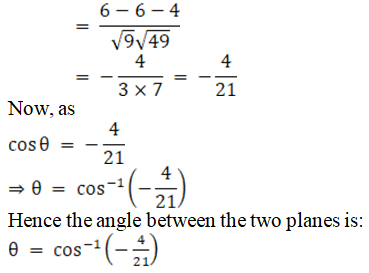iii.

Solution: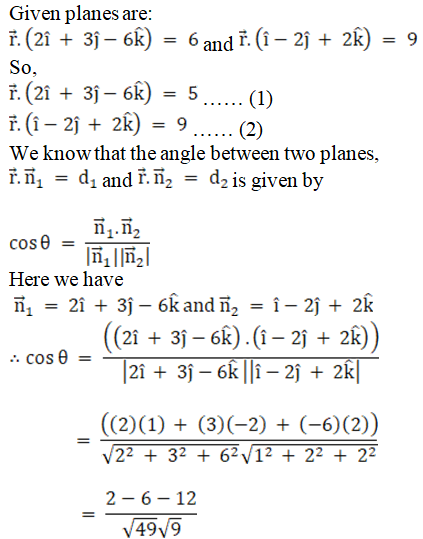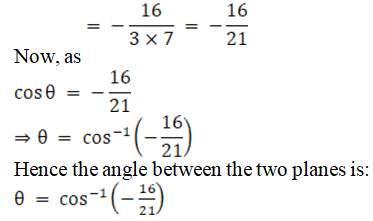Q2. i

Solution:

Given planes are:

2x – y + z = 4 and x + y + 2z = 3

We know that angle between two planes,

a1x + b1y + c1z + d1 = 0 and

a2x + b2y + c2z + d2 = 0 is given as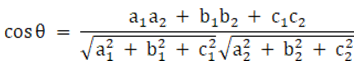So here we have,

a1 = 2, b1 = – 1, c1 = 1

a2 = 1, b2 = 1, c2 = 2

Now let us substitute the values in above expression, we get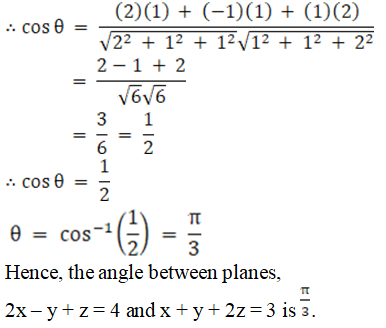ii.

Solution:

Given planes are:

x + y – 2z = 3 and 2x – 2y + z = 5

We know that angle between two planes,

a1x + b1y + c1z + d1 = 0

a2x + b2y + c2z + d2 = 0 is given asSo here we have,

a1 = 1, b1 = 1, c1 = – 2

a2 = 2, b2 = – 2, c2 = 1

Now let us substitute the values in above expression, we get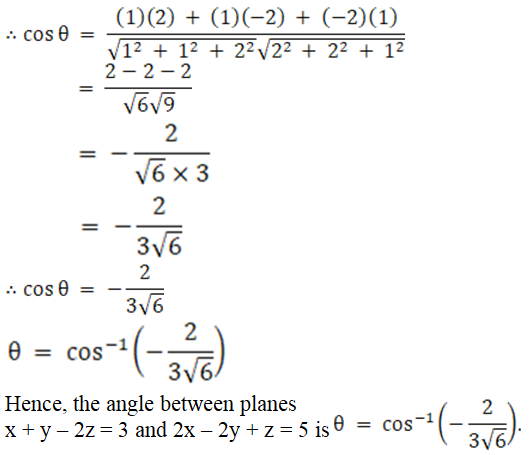iii.

Solution:

Given planes are:

x – y + z = 5 and x + 2y + z = 9

We know that angle between two planes,

a1x + b1y + c1z + d1 = 0

a2x + b2y + c2z + d2 = 0 is given asSo here we have,

a1 = 1, b1 = – 1, c1 = 1

a2 = 1, b2 = 2, c2 = 1

Now let us substitute the values in above expression, we get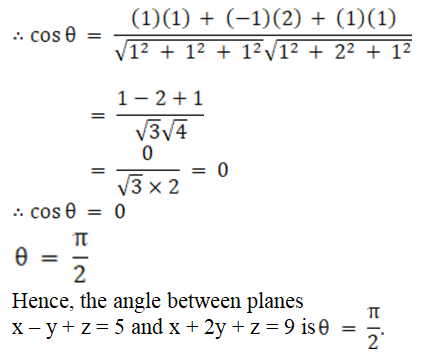iv.

Solution:

Given planes are:

2x – 3y + 4z = 1 and – x + y = 4

We know that angle between two planes,

a1x + b1y + c1z + d1 = 0

a2x + b2y + c2z + d2 = 0 is given asSo here we have,

a1 = 2, b1 = – 3, c1 = 4

a2 = – 1, b2 = 1, c2 = 0

Now let us substitute the values in above expression, we get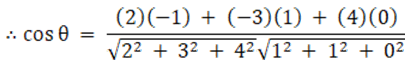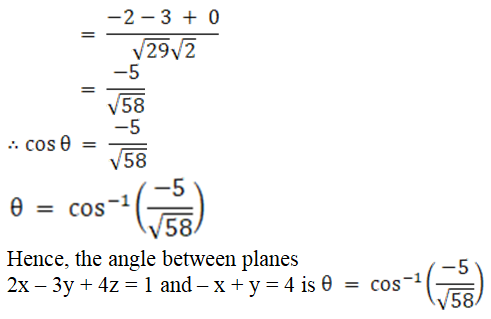v.

Solution:

Given planes are:

2x + y – 2z = 5 and 3x – 6y – 2z = 7

We know that angle between two planes,

a1x + b1y + c1z + d1 = 0

a2x + b2y + c2z + d2 = 0 is given asSo here we have,

a1 = 2, b1 = 1, c1 = – 2

a2 = 3, b2 = – 6, c2 = – 2

Now let us substitute the values in above expression, we get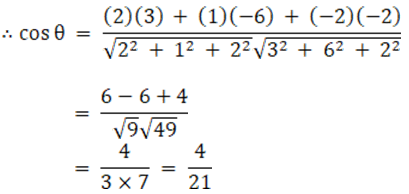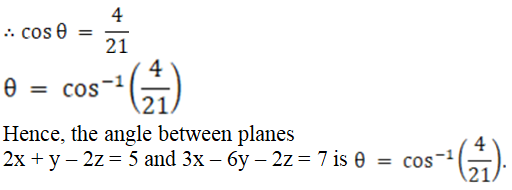Q3.i.

Solution: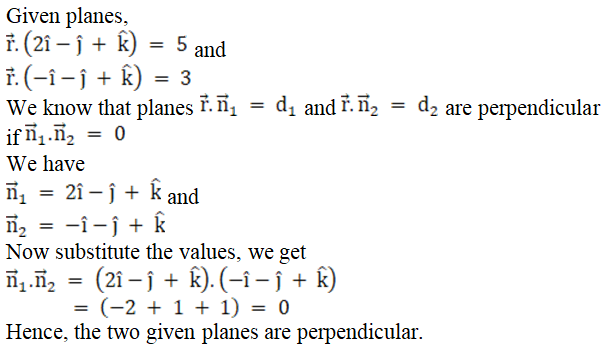ii.

Solution:

Given planes,

x – 2y + 4z = 10 and

18x + 17y + 4z = 49

We know that planes

a1x + b1y + c1z + d1 = 0 and

a2x + b2y + c2z + d2 = 0 are at right angles if,

a1a2 + b1b2 + c1c2 = 0 …… (1)

Now we have,

a1 = 1, b1 = – 2, c1 = 4 and

a2 = 18, b2 = 17, c2 = 4

Using equation (1) we have,

a1a2 + b1b2 + c1c2 = (1) (18) + (– 2) (17) + (4) (4)

= 18 – 34 + 16 = 0

Hence, the planes are at right angle to each other.

Q4.i.

Solution: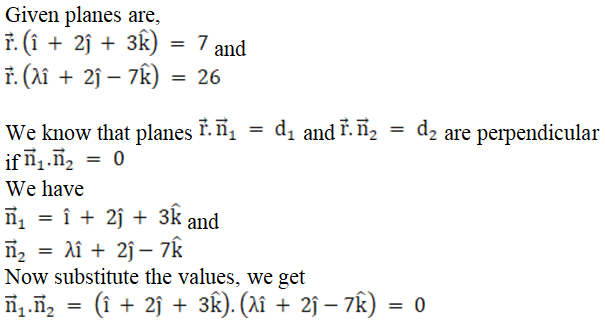So, (λ + 4 – 21) = 0

λ = 21 – 4

= 17

Hence, for λ = 17 the given planes are perpendicular.

ii.

Solution:

Given planes are,

2x – 4y + 3z = 5 and

x + 2y + λz = 5

We know that planes

a1x + b1y + c1z + d1 = 0 and

a2x + b2y + c2z + d2 = 0 are at right angles if,

a1a2 + b1b2 + c1c2 = 0 …… (1)

We have,

a1 = 2, b1 = – 4, c1 = 3 and

a2 = 1, b2 = 2, c2 = λ

Let us substitute the values in equation (1), we get

a1a2 + b1b2 + c1c2 => (2) (1) + (– 4) (2) + (3) (λ) = 0

=> 2 – 8 + 3λ = 0

6 = 3λ

2 = λ

Hence, for λ = 2 the given planes are perpendicular.

iii.

Solution:

Given planes are,

3x – 6y – 2z = 7 and

2x + y – λz = 5

We know that planes

a1x + b1y + c1z + d1 = 0 and

a2x + b2y + c2z + d2 = 0 are at right angles if,

a1a2 + b1b2 + c1c2 = 0 …… (1)

We have,

a1 = 3, b1 = – 6, c1 = – 2 and

a2 = 2, b2 = 1, c2 = – λ

Let us substitute the values in equation (1), we get

a1a2 + b1b2 + c1c2 = (3) (2) + (– 6) (1) + (– 2) (– λ) = 0

=> 6 – 6 + 2λ = 0

0 = – 2λ

0 = λ

Hence, for λ = 0 the given planes are perpendicular to each other.

Q5.

Solution:

We know that solution of a plane passing through (x1, y1, z1) is given as:

a(x – x1) + b(y – y1) + c(z – z1) = 0

The required plane passes through (– 1, – 1, 2),

So the equation of plane is

a(x + 1) + b(y + 1) + c(z – 2) = 0

⇒ ax + by + cz = 2c – a – b …… (1)

Now, the required plane is also perpendicular to the planes,

3x + 2y – 3z = 1 and

5x – 4y + z = 5

We know that planes

a1x + b1y + c1z + d1 = 0 and

a2x + b2y + c2z + d2 = 0 are at right angles if,

a1a2 + b1b2 + c1c2 = 0 …… (2)

Using equation (2) we have,

3a + 2b – 3c = 0 …… (3)

5a – 4b + c = 0 …… (4)

Now let us solve equation (3) and (4) we get,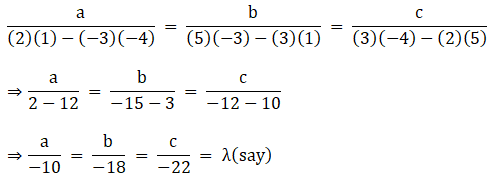So,

a = – 10λ, b = – 18λ, c = – 22λ

Now substitute the values of a, b, c in equation (1) we get,

(– 10λ)x + (– 18λ)y + (– 22λ)z = 2(– 22)λ – (– 10λ) – (– 18λ)

– 10λx – 18λy – 22λz = – 44λ + 10λ + 18λ

– 10λx – 18λy – 22λz = – 16λ

Divide both sides by (– 2λ), we get

5x + 9y + 11z = 8

Hence, the required equation of the plane is 5x + 9y + 11z = 8.